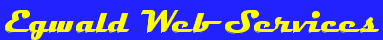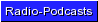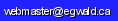Egwald Mathematics — Nonlinear Dynamics by Elmer G. Wiens Egwald's popular web pages are provided without cost to users. Please show your support by joining Egwald Web Services as a Facebook Fan:Follow Elmer Wiens on Twitter:One Dimensional Dynamics and Bifurcations One Dimensional Continuous Time Models. Continuous time nonlinear dynamic processes use differential equations to describe the dynamics of the system. 1. Introduction to nonlinear dynamics using the model of population dynamics. 2. The well-known logistic equation of population dynamics. 3. The general form of a nonlinear differential equation. 4. The local stability of a nonlinear differential equation about a fixed point using linear stability analysis. 5. The major categories of one dimensional continuous time bifurcations: 6. Examples of bifurcation from economic theory: A saddle-node bifurcation in the labour market A transcritical bifurcation in the neoclassical economic growth model A pitchfork bifurcation in the Kaldor business cycle model One Dimensional Discrete Time Models. Discrete time, nonlinear dynamic processes use difference equations to describe the dynamics of the system. 1. Introduction to discrete time, nonlinear dynamic models. 2. The general form of a nonlinear difference equation. 3. The local stability of a nonlinear difference equation about a fixed point using linear stability analysis. 4. The higher order maps of a one dimensional difference equation. 5. The major categories of one dimensional discrete time bifurcations: The one-dimensional, quadratic, logistic map can be used to demonstrate complex, dynamic phenomena that also occur in chaos theory and higher dimensional discrete time systems. 1. Introduction to the logistic map. 2. The linearized stability of the logistic map. 3. The bifurcation analysis of the logistic map. 4. The period doubling bifurcations of the logistic map. 5. The period doubling cascade path to chaos. 6. The logistic map orbit diagram. 7. The third order map, f3. 8. Third order map period doubling cascade path to chaos. 9. Click to pop a new window with some interactive logistic map diagrams. 10. Definitions of chaos. The Trygve Haavelmo growth model provides an example of the different dynamical behaviours arising from equivalent models expressed as either differential or difference equations. While the solution trajectories of the continuous time version of the model converge to its fixed point, the solution trajectories of the discrete time version may exhibit chaotic behaviour. 1. In the continuous time version of the model, solution trajectories of the differential equation governing the growth of employment converge to a stable fixed point. 2. The phase diagrams show the solution trajectories for various values of the parameter α of the model's differential equation. 3. In the discrete time version of the model, solution trajectories may follow periodic cycles and eventual exhibit chaotic behaviour as the parameter α of the model's difference equation increase. 4. The phase diagrams show the solution trajectories for various values of the parameter α of the model's difference equation. 5. The bifurcation diagram shows the period doubling cascade path to chaos.Copyright © Elmer G. Wiens:   Egwald Web ServicesAll Rights Reserved.    Inquiries